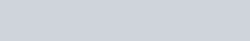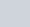Week 0171 : 2nd October 2023

Search

# DATUM

The average of the scores obtained on a board (i.e. add up all the scores and divide by the total number of scores). In most sizes of field, the calculation ignores one or more of the top and bottom scores to give a more representative score. The purpose of calculating the datum is so that you can compare an individual pair’s score with the datum and convert it to IMPs.

As an example, suppose the scores on a board are +1100, +620, +620, +620, +140 and -100. Ignoring the top score (+1100) and the bottom score (-100), the total score is 2000. Dividing this by 4 gives 500, which is the datum.